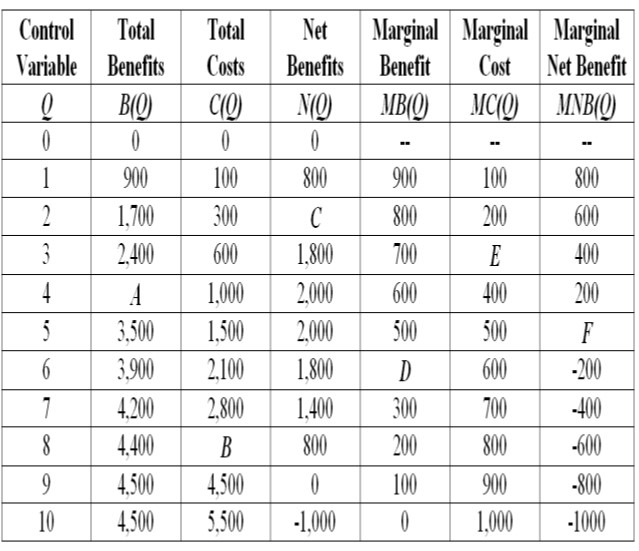# MCR001 Economics

```{`MCR001 Economics
TRIMESTER 2 2020 FINAL EXAMINATION
`}```

There are four compulsory questions – you are required to answer all four.

Question 1 (15 marks)

1. What are the environments in which businesses operate? (5 marks)
2. Examine the management challenges they face in all these environments. (5 marks)
3. Demonstrate with examples how the firms face these challenges posed by the COVID crisis. (5 marks)

Question 2 (15 marks)

Let the demand for some item is represented as D = 30 – 2p + 0.04 Y + 4 s where D is the quantity demanded in units a year, p is the price of the item in dollars per unit, Y is the buyer’s income in thousands of dollars a year, and s is the value of another factor such as preference.

1. Suppose Y = 100 and s = 0.5, describe in words the relationship between quantity demanded and price. (4 marks)
2. Examine the effect of changes in prices and changes in income on the demand curve. Clearly explain the differences in the quantity demanded due to these changes. (6 marks)
3. What are some of the questions you will ask as an advisor to the owner of firm facing such demand conditions? (5 marks)

Question 3 (15 marks)

A business you are working for faces the following internal business scenario:1. What is the total benefit associated with producing four units of the control variable, Q (identified as point A in the above table)? (1mark)
2. What is the total cost associated with producing eight units of the control variable, Q (identified as point B in the above table)? (1mark)
3. What is the net benefit associated with producing two units of the control variable, Q (identified as point C in the above table)? (2 marks)
4. What is the marginal benefit associated with producing six units of the control variable,
5. What is the marginal cost associated with producing three units of the control variable,

Q (identified as point D in the above table)? (2marks)

Q (identified as point E in the above table)? (2marks)

6. What is the marginal net benefit associated with producing five units of the control variable,

Q (identified as point F in the above table)? (2marks)

7. How many units will you advise the firm to produce? Make explicit your reasons. (5 marks)

Question 4 (15 marks)

The coronavirus pandemic is leading to several management challenges in all echelons of the economic environment. These challenges are further compounded depending on the extent of the openness of the economy.

1. List the management challenges in an open economy such as Australia. (Hint: Examine pre COVID, during COVID and post COVID) (3 marks)
2. Examine some of the business strategies you would suggest meeting the management challenges if you were managing each of the following: (12 marks)